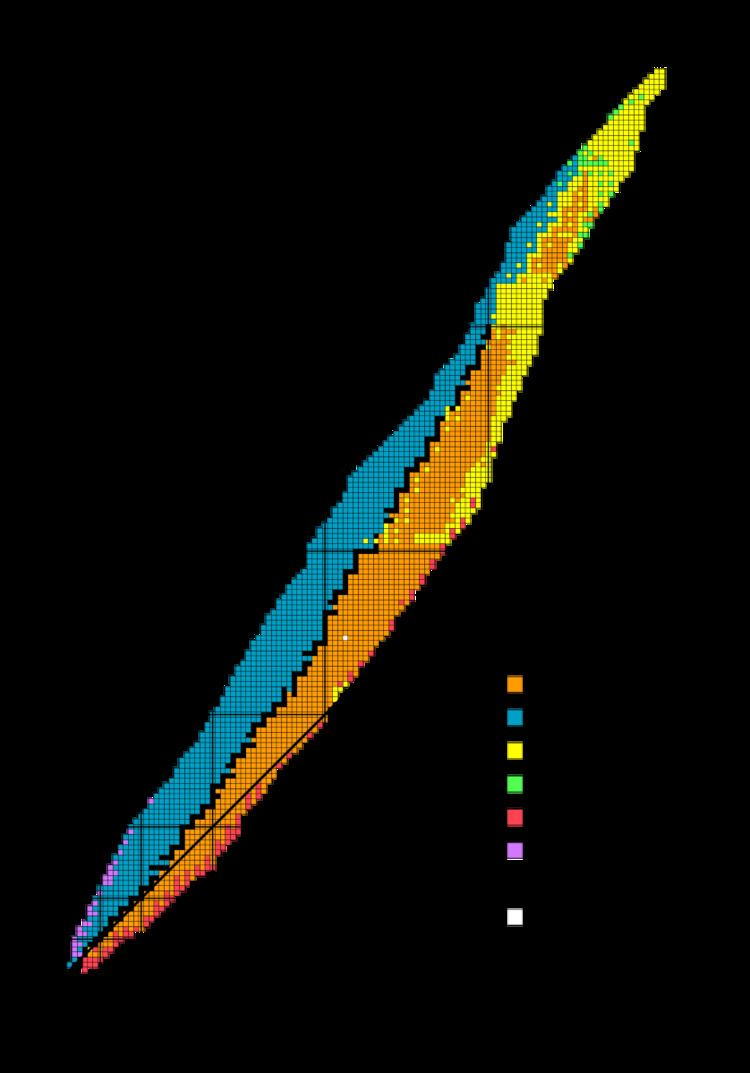# Beta decay stable isobars

Updated on
Covid-19Beta-decay stable isobars are the set of nuclides which cannot undergo beta decay, that is, the transformation of a neutron to a proton or a proton to a neutron within the nucleus. A subset of these nuclides are also stable with regards to double beta decay or theoretically higher simultaneous beta decay, as they have the lowest energy of all nuclides with the same mass number.

## Contents

This set of nuclides is also known as the line of beta stability, a term already in common use in 1965. This line lies along the bottom of the nuclear valley of stability.

## Introduction

The line of beta stability can be defined mathematically by finding the nuclide with the greatest binding energy for a given mass number, by a model such as the classical semi-empirical mass formula developed by C. F. Weizsäcker. These nuclides are local maxima in terms of binding energy for a given mass number.

All odd mass numbers have only one beta decay stable nuclide.

Among even mass number, seven (96, 124, 130, 136, 148, 150, 154) have three beta-stable nuclides. None have more than three, all others have either one or two.

• From 2 to 34, all have only one.
• From 36 to 72, only nine (36, 40, 46, 48, 50, 54, 58, 64, 70) have two, and the remaining 11 have one.
• From 74 to 122, only three (88, 90, 118) have one, and the remaining 22 have two.
• From 124 to 154, only one (140) has one, six have three, and the remaining 9 have two.
• From 156 to 260, only seventeen have one, and the remaining 36 have two.
• All primordial nuclides are beta decay stable, with the exception of 40K, 50V, 87Rb, 113Cd, 115In, 138La, 176Lu, and 187Re. In addition, 123Te and 180mTa have not been observed to decay, but are believed to undergo beta decay with an extremely long half-life (over 1015 years). All elements up to and including nobelium, except technetium and promethium, are known to have at least one beta-stable isotope.

## List of known beta-decay stable isobars

Theoretically predicted or experimentally observed double beta-decay (if not dominated by alpha decay or spontaneous fission) is shown by arrows, i.e. arrows point towards the lightest-mass isobar. There are currently known to be 358 beta-decay stable nuclides.

All beta-decay stable nuclides with A ≥ 209 were observed to decay by alpha decay except some where spontaneous fission dominates.

261No, 263Lr, 264No, 264Rf, 265Lr, 269Db have not yet been synthesized; the synthesis of 260Fm, 262No, 266No, 266Rf, 268Rf, 270Rf is unconfirmed.

Further predicted beta-decay stable isobars are 272Sg, 275Bh, 278Hs, 281Mt, 284Ds, 287Rg, 290Cn, 293Uut, 296Fl, 299Uup, 302Lv, and 305Uus.

## Beta decay toward minimum mass

Beta decay generally causes isotopes to decay toward the isobar with the lowest mass (highest binding energy) with the same mass number, those not in italics in the table above. Thus, those with lower atomic number and higher neutron number than the minimum-mass isobar undergo beta-minus decay, while those with higher atomic number and lower neutron number undergo beta-plus decay or electron capture. However, there are four nuclides that are exceptions, in that the majority of their decays are in the opposite direction: